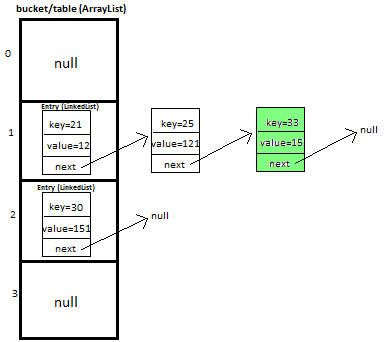### How Integer is used as key in Hashmap in java

Let’s understand in detail how Integer is used as key in Hashmap.

Here I will try to explain how Integer is internally used as key in HashMap in Java Api.

Initially, we have bucket of capacity=4. (all indexes of bucket i.e. 0,1,2,3 are pointing to null).
Note: Initial capacity provided by Java Api is different.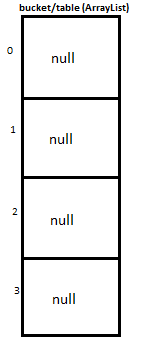Let’s put first key-value pair in HashMap-
Key=21, value=12
newEntry Object will be formed like this >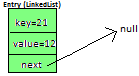We will calculate hash by using our hash(K key) method - in this case it returns
key/capacity= 21%4= 1.
So, 1 will be the index of bucket on which newEntry object will be stored.
We will go to 1st index as it is pointing to null we will put our newEntry object there.

At completion of this step, our HashMap will look like this-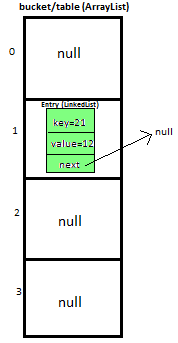Let’s put second key-value pair in HashMap-
Key=25, value=121
newEntry Object will be formed like this >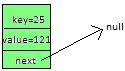We will calculate hash by using our hash(K key) method - in this case it returns
key/capacity= 25%4= 1.
So, 1 will be the index of bucket on which newEntry object will be stored.
We will go to 1st index, it contains entry with key=21, we will compare two keys(i.e. compare 21 with 25 by using equals method), as two keys are different we check whether entry with key=21’s next is null or not, if next is null we will put our newEntry object on next.

At completion of this step our HashMap will look like this-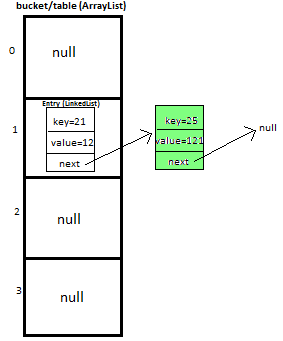Let’s put third key-value pair in HashMap-
Key=30, value=151
newEntry Object will be formed like this >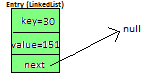We will calculate hash by using our hash(K key) method - in this case it returns
key/capacity= 30%4= 2.
So, 2 will be the index of bucket on which newEntry object will be stored.
We will go to 2nd  index as it is pointing to null we will put our newEntry object there.

At completion of this step, our HashMap will look like this-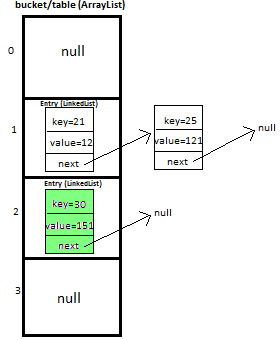Let’s put fourth key-value pair in HashMap-
Key=33, value=15
Entry Object will be formed like this >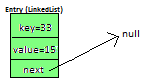We will calculate hash by using our hash(K key) method - in this case it returns
key/capacity= 33%4= 1,
So, 1 will be the index of bucket on which newEntry object will be stored.

We will go to 1st index -
>it contains entry with key=21, we will compare two keys (i.e. compare 21 with 33 by using equals method, as two keys are different,  proceed to next  of entry with key=21 (proceed only if next is not null).
>now, next contains entry with key=25, we will compare two keys (i.e. compare 25 with 33 by using equals method, as two keys are different,  now next of entry with key=25 is pointing to null so we won’t proceed further, we will put our newEntry object on next.

At completion of this step our HashMap will look like this-